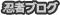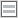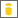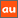# 0除算に関するアナウンスメントを公開しています

The purpose of this site is to publish announcements on the theory of division by zero

## Announcement 380: What is the zero?

Institute of Reproducing Kernels
Kawauchi-cho, 5-1648-16,
Kiryu 376-0041, Japan
August 21, 2017

1 What is the zero?

The zero $0$ as the complex number or real number is given clearly by the axions by the complex number field and real number field.

For this fundamental idea, we should consider the Yamada field  containing the division by zero. The Yamada field and the division by zero calculus will arrange our mathematics, beautifully and completely; this will be our natural and complete mathematics.

2 Double natures of the zero $z=0$

The zero point $z=0$ represents the double natures; one is the origin at the starting point and another one is a representation of the point at infinity. One typical and simple example is given by $e^0 = 1,0$, two values.  God loves  two.

3 Standard value

The zero is a center and stand point $($or bases, a standard value$)$ of the coordinates - here we will consider our situation on the complex or real 2 dimensional spaces. By stereographic  projection mapping or the Yamada field, the point at infinity $1/0$ is represented by zero. The origin of the coordinates and the point at infinity correspond each other.
As the standard value, for the point $\omega_n = \exp \left(\frac{\pi}{n}i\right)$  on the unit circle $|z|=1$ on the complex $z$-plane is,  for $n = 0$:
\begin{equation}
\omega_0 = \exp \left(\frac{\pi}{0}i\right)=1, \quad  \frac{\pi}{0} =0.
\end{equation}
For the mean value
$$M_n = \frac{x_1 + x_2 +... + x_n}{n},$$
we have
$$M_0 = 0 = \frac{0}{0}.$$

4 Fruitful world

For example, for very and very general partial differential equations, if the coefficients or terms are zero, then we have some simple differential equations and the extreme case is all the terms are zero; that is, we have trivial equations $0=0$; then its solution is zero. When we consider the converse, we see that the zero world is a  fruitful one and it means some vanishing world. Recall Yamane phenomena $($$)$, the vanishing result is very simple zero, however, it is the result from some fruitful world. Sometimes, zero means void or nothing world, however, it will show some changes as in the Yamane phenomena.

5 From $0$ to $0$; $0$ means all and all are $0$

As we see from our life figure $($$)$, a story starts from the zero and ends with the zero. This will mean that $0$ means all and all are $0$. The zero is a mother or an  origin of all.

6 Impossibility

As the solution of the simplest equation
\begin{equation}\tag{6.1}
ax =b
\end{equation}we have $x=0$ for $a=0, b\ne 0$ as the standard value, or the Moore-Penrose generalized inverse. This will mean in a sense, the solution does not exist; to solve the equation $($6.1$)$ is impossible. We saw for different parallel lines or different parallel planes, their common points are the origin. Certainly they have the common points of the point at infinity and the point at infinity is represented by zero. However, we can understand also that they have no solutions, no common points, because the point at infinity is an ideal point.
Of course. we can consider the equation $($6.1$)$  even the case $a=b=0$ and then we have the solution $x=0$ as we stated.
We will consider the simple differential equation
\begin{equation}
m\frac{d^2x}{dt^2} =0,  m\frac{d^2y}{dt^2} =-mg
\end{equation}
with the initial conditions, at $t =0$
\begin{equation}
\frac{dx}{dt} = v_0 \cos \alpha , \frac{d^2x}{dt^2} = \frac{d^2y}{dt^2}=0.
\end{equation}Then,  the highest high $h$, arriving time $t$, the distance $d$ from the starting point at the origin to the point $y(2t) =0$ are given by
\begin{equation}
h = \frac{v_0 \sin^2 \alpha}{2g},  d= \frac{v_0\sin \alpha}{g}
\end{equation}and
\begin{equation}
t= \frac{v_0 \sin \alpha}{g}.
\end{equation}
For the case $g=0$, we have $h=d =t=0$. We considered the case that they are the infinity; however, our mathematics means zero, which shows impossibility.
These phenomena were looked many cases on the universe; it seems that God does not like the infinity.

References
 M. Kuroda, H. Michiwaki, S. Saitoh, and M. Yamane, New meanings of the division by zero and interpretations on $100/0=0$ and on $0/0=0$, Int. J. Appl. Math.  27 $($2014$)$, no 2, pp. 191-198,  DOI: 10.12732/ijam.v27i2.9.
 H. Michiwaki, S. Saitoh,  and  M.Yamada, Reality of the division by zero $z/0=0$.  IJAPM  International J. of Applied Physics and Math.  6 $($2015$)$, 1--8. http://www.ijapm.org/show-63-504-1.html
 T. Matsuura and S. Saitoh, Matrices and division by zero $z/0=0$, Advances in Linear Algebra & Matrix Theory, 6 $($2016$)$, 51-58. http://dx.doi.org/10.4236/alamt.2016.62007 http://www.scirp.org/journal/alamt
 H.  Michiwaki, H. Okumura, and S. Saitoh, Division by Zero $z/0 = 0$ in Euclidean Spaces.  International Journal of Mathematics and Computation 28 $($2017$)$; Issue  1, 2017), 1-16.
 H. Okumura, S. Saitoh and T. Matsuura, Relations of   $0$ and  $\infty$, Journal of Technology and Social Science $($JTSS$)$, 1$($2017$)$,  70-77.
 H. G. Romig, Discussions: Early History of Division by Zero, American Mathematical Monthly, Vol. 31, No. 8. $($Oct., 1924$)$, pp. 387-389.
 S. Saitoh, Generalized inversions of Hadamard and tensor products for matrices,  Advances in Linear Algebra \& Matrix Theory.  4 $($2014$)$, no. 2,  87--95. http://www.scirp.org/journal/ALAMT/
 S. Saitoh, A reproducing kernel theory with some general applications, Qian,T./Rodino,L.$($eds.$)$: Mathematical Analysis, Probability and Applications - Plenary Lectures: Isaac 2015, Macau, China, Springer Proceedings in Mathematics and Statistics,   177$($2016$)$, 151-182 $($Springer$)$.
 S.-E. Takahasi, M. Tsukada and Y. Kobayashi,  Classification of continuous fractional binary operations on the real and complex fields,  Tokyo Journal of Mathematics, 38$($2015$)$, no. 2, 369-380.
 Announcement 179 $($2014.8.30$)$: Division by zero is clear as z/0=0 and it is fundamental in mathematics.
 Announcement 185 $($2014.10.22$)$: The importance of the division by zero $z/0=0$.
 Announcement 237 $($2015.6.18$)$:  A reality of the division by zero $z/0=0$ by  geometrical optics.
 Announcement 246 $($2015.9.17$)$: An interpretation of the division by zero $1/0=0$ by the gradients of lines.
 Announcement 247 $($2015.9.22$)$: The gradient of y-axis is zero and $\tan (\pi/2) =0$ by the division by zero $1/0=0$.
 Announcement 250 $($2015.10.20$)$: What are numbers? -  the Yamada field containing the division by zero $z/0=0$.
 Announcement 252 $($2015.11.1$)$: Circles and curvature - an interpretation by Mr. Hiroshi Michiwaki of the division by zero $r/0 = 0$.
 Announcement 281 $($2016.2.1$)$: The importance of the division by zero $z/0=0$.
 Announcement 282 $($2016.2.2$)$: The Division by Zero $z/0=0$ on the Second Birthday.
 Announcement 293 $($2016.3.27$)$:  Parallel lines on the Euclidean plane from the viewpoint of division by zero 1/0=0.
 Announcement 300 $($2016.05.22$)$: New challenges on the division by zero z/0=0.
 Announcement 326 $($2016.10.17$)$: The division by zero z/0=0 - its impact to human beings through education and research.
 Announcement 352 $($2017.2.2$)$:   On the third birthday of the division by zero z/0=0.
 Announcement 354 $($2017.2.8$)$: What are $n = 2,1,0$ regular polygons inscribed in a disc? -- relations of $0$ and infinity.
 Announcement 362$($2017.5.5$)$:   Discovery of the division by zero as $0/0=1/0=z/0=0$.

PR

### コメント

お名前 Black LimeGreen SeaGreen Teal FireBrick Tomato IndianRed BurlyWood SlateGray DarkSlateBlue LightPink DeepPink DarkOrange Gold DimGray Silverプロフィール

ＨＮ：

(01/17)
(01/14)
(01/12)
(01/04)
(01/03)